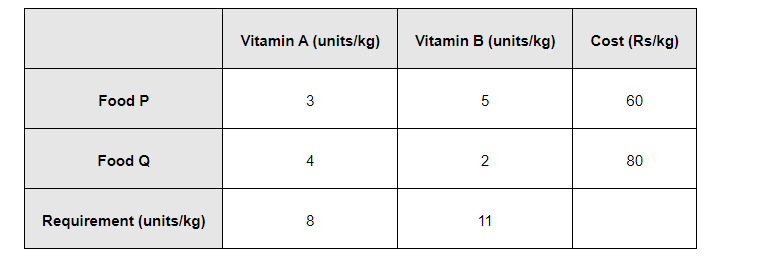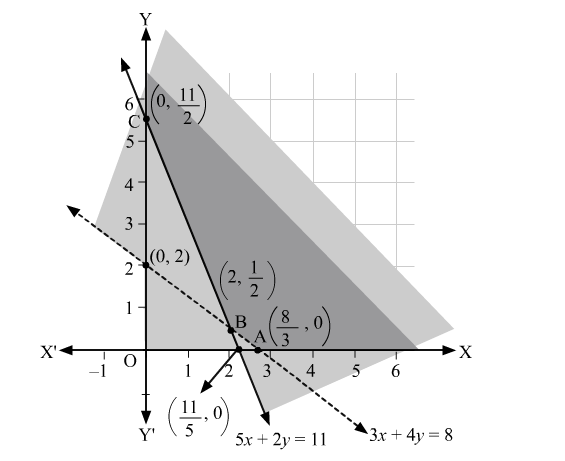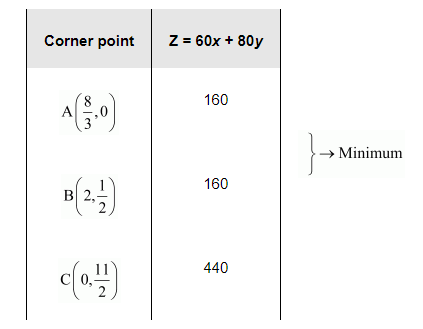# Reshma wishes to mix two types of food P and Q in such a way that the vitamin contents of the mixture contain at least 8 units of vitamin A and 11 units of vitamin B

Question:

Reshma wishes to mix two types of food P and Q in such a way that the vitamin contents of the mixture contain at least 8 units of vitamin A and 11 units of vitamin B. Food P costs Rs 60/kg and Food Q costs Rs 80/kg. Food P contains 3 units /kg of vitamin A and 5 units /kg of vitamin B while food Q contains 4 units /kg of vitamin A and 2 units /kg of vitamin B. Determine the minimum cost of the mixture?

Solution:

Let the mixture contain x kg of food P and y kg of food Q. Therefore,

$x \geq 0$ and $y \geq 0$

The given information can be compiled in a table as follows.The mixture must contain at least 8 units of vitamin A and 11 units of vitamin B. Therefore, the constraints are

$3 x+4 y \geq 8$

$5 x+2 y \geq 11$

Total cost, Z, of purchasing food is, Z $=60 x+80 y$

The mathematical formulation of the given problem is

Minimise $Z=60 x+80 y \ldots$

subject to the constraints,

$3 x+4 y \geq 8 \ldots(2)$

$5 x+2 y \geq 11 \ldots(3)$

$x, y \geq 0 \ldots(4)$

The feasible region determined by the system of constraints is as follows.It can be seen that the feasible region is unbounded.

The corner points of the feasible region are $\mathrm{A}\left(\frac{8}{3}, 0\right), \mathrm{B}\left(2, \frac{1}{2}\right)$, and $\mathrm{C}\left(0, \frac{11}{2}\right)$.

The values of Z at these corner points are as follows.As the feasible region is unbounded, therefore, 160 may or may not be the minimum value of Z.

For this, we graph the inequality, $60 x+80 y<160$ or $3 x+4 y<8$, and check whether the resulting half plane has points in common with the feasible region or not.

It can be seen that the feasible region has no common point with $3 x+4 y<8$

Therefore, the minimum cost of the mixture will be Rs 160 at the line segment joining the points $\left(\frac{8}{3}, 0\right)$ and $\left(2, \frac{1}{2}\right)$.